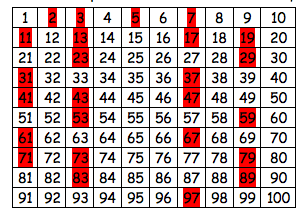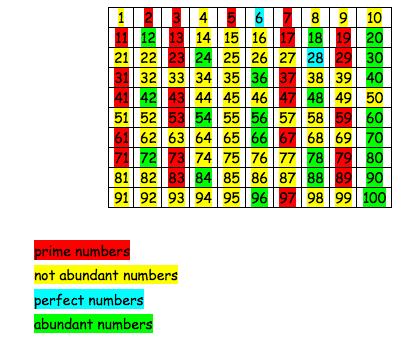#### You may also like### Pebbles

Place four pebbles on the sand in the form of a square. Keep adding as few pebbles as necessary to double the area. How many extra pebbles are added each time?### Bracelets

Investigate the different shaped bracelets you could make from 18 different spherical beads. How do they compare if you use 24 beads?### Sweets in a Box

How many different shaped boxes can you design for 36 sweets in one layer? Can you arrange the sweets so that no sweets of the same colour are next to each other in any direction?

# Abundant Numbers

##### Age 7 to 11Challenge Level

Georgia, from Holy Trinity School, found two more abundant numbers:

$36$: total is $55$ ($1+2+3+4+6+9+12+18$)
$70$: total is $74$ ($1+2+5+7+10+14+35$)

Rachael, Jamie, Heledd, Sian, Dafydd, Tom, Edward and Isaac from Ysgol Bryncrug clearly worked hard on this problem.  They told us:

We decided to find out which of the numbers from $1$ to $100$ are abundant numbers.
We decided that prime numbers are not abundant numbers:
$2, 3, 5, 7, 11, 13, 17, 19, 23, 29, 31, 37, 41, 43, 47, 53, 59, 61, 67, 71, 73, 79, 83, 89, 97$
because without themselves $1$ is their only factor.

We crossed out all the prime numbers from our $100$ square:Then we looked at all the remaining numbers. Here is an example:

$27 = 1\times27$, $3\times9$
So the factors of $27$ are: $1, 3, 9, 27$
The sum of its factors without itself is: $1+3+9 = 13$

$13$ is less than $27$ therefore $27$ is not an abundant number

And another example:
$48 = 1\times48$, $2\times24$, $3\times16$, $4\times12$, $6\times8$
So the factors of $48$ are: $1, 2, 3, 4, 6, 8, 12, 16, 24, 48$.
The sum of its factors without itself is:
$1+2+3+4+6+8+12+16+24 = 76$

$76$ is greater than $48$ therefore $48$ is an abundant number

Something different happened with $6$:
$6 = 1\times6$, $2\times3$
So the factors of $6$ are: $1, 2, 3$ and $6$
The sum of its factors without itself is: $1+2+3 = 6$

$6$ is equal to $6$!!

This also happened with $28$: $1+2+4+7+14 = 28$

Edward wanted to find out what we call a number where the sum of its factors (without itself) is equal to the number. He asked his Dad and he told him that it is called a PERFECT NUMBER.
He also found out that the next perfect number after $28$ is $496$. Then $8128$!!

We continued marking the numbers on the $100$ square:There are twenty two abundant numbers on our $100$ square.

We had an email from a teacher at St Joseph's Primary School in Plymouth, who sent in the following;

I tackled the abundant numbers problem with some of my Year 6 pupils which provided a really high level of challenge for the boys. Having thought they found the answer and solution, they were faced with a couple of anomalies which they then investigated further.

Their solution to identifying whether a number is an abundant number without having to add the numbers up was that if the number has 6 factors or fewer, it is an abundant number. If the number has more than 6 factors, then it won't be abundant number.

Having looked through your solutions, I thought that this discovery after quite a lengthy investigation was worthy of being on your website. The boys were Elias and Xavi.

We requested more information and the teacher emailed back what the boys said;

We started quite randomly by choosing 36 and 96 and realised that they were both abundant numbers but then we tried with 44 and that wasn't an abundant number (22 + 11 + 4 + 2 + 1 = 40). This meant that our prediction of even numbers being abundant numbers was wrong.

We then started at 10 and realised this wasn't an abundant number (5 + 2 + 1 = 8)
We tried 11 but quickly realised that no prime number could be an abundant number.
Then we tried 12 and this was an abundant number  (6 + 4 + 3 + 2 + 1 =16)
We looked at 14 and it wasn't. 15 wasn't (5 + 3 + 1 = 9). 16 wasn't (4 + 1 = 5). 18 was (9 + 6 + 3 + 2 + 1 = 21).

Using these answers, we saw that an abundant number was any number that had 6 or more factors. We could then tell before adding anything up whether a number would be abundant. We knew that 40 would be abundant because it is 20 + 10 + 8 + 5 + 4 + 2 + 1 = 50.

Thank you all very much for your contribitions. Enjoy further mathematical journeys exploring number. Well done!